NEET  >  NEET Previous Year Questions (2014-22): Moving Charges & Magnetism

# NEET Previous Year Questions (2014-22): Moving Charges & Magnetism - Notes | Study Physics Class 12 - NEET

 1 Crore+ students have signed up on EduRev. Have you?

Q.1. A long solenoid of radius 1 mm has 100 turns per mm. If 1 A current flows in the solenoid, the magnetic field strength at the center of the solenoid is    

(1) 12.56 × 10–2 T
(2) 12.56 × 10–4 T
(3) 6.28 × 10–4 T
(4) 6.28 × 10–2 T
Sol. We know, the magnetic field at center of solenoid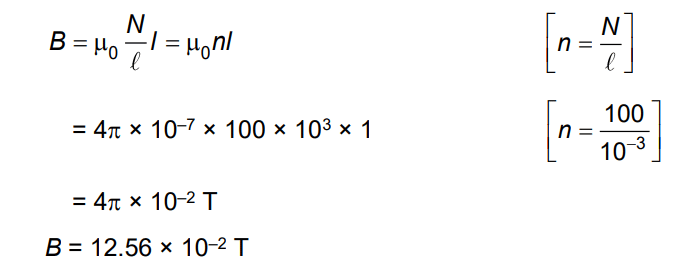Q.2. From Ampere’s circuital law for a long straight wire of circular cross-section carrying a steady current, the variation of magnetic field in the inside and outside region of the wire is
(1) A linearly increasing function of distance upto the boundary of the wire and then linearly decreasing for the outside region.
(2) A linearly increasing function of distance r upto the boundary of the wire and then decreasing one with 1/r dependence for the outside region.
(3) A linearly decreasing function of distance upto the boundary of the wire and then a linearly increasing one for the outside region.
(4) Uniform and remains constant for both regions.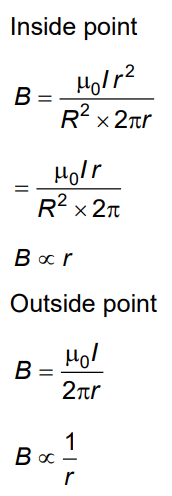Q.3. Given below are two statements

Statement I: Biot-Savart’s law gives us the expression for the magnetic field strength of an infinitesimal current element (Idl) of a current-carrying conductor only.
Statement II: Biot-Savart’s law is analogous to Coulomb’s inverse square law of charge q, with the former being related to the field produced by a scalar source, Idl while the latter being produced by a vector source, q. In light of the above statements choose the most appropriate answer from the options given below       (2022)

(1) Both Statement I and Statement II are incorrect
(2) Statement I is correct and Statement II is incorrect
(3) Statement I is incorrect and Statement II is correct
(4) Both Statement I and Statement II are correct

Sol. According to Biot-Savart’s law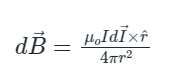which is applicable for infinitesimal element. It is analogous to Coulomb’s law, where IdI is vector source and electric field is produced by scalar source q. Here, statement I is correct and statement II is incorrect.

Q.4. A thick current-carrying cable of radius 'R' carries current 'I' uniformly distributed across its cross-section. The variation of magnetic field B(r) due to the cable with the distance 'r' from the axis of the cable is represented by:      (2021)
A: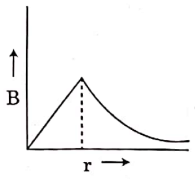B: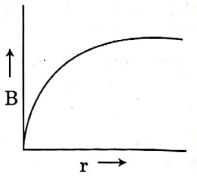C: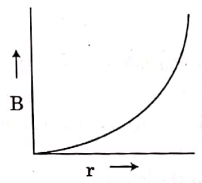D: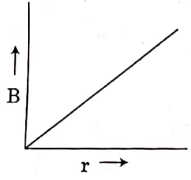Ans: AInside a current carrying cylindrical conductorQ.5. An infinitely long straight conductor carries a current of 5 A as shown. An electron is moving with a speed of 10m/s parallel to the conductor. The perpendicular distance between the electron and the conductor is 20 cm at an instant. Calculate the magnitude of the force experienced by the electron at that instant.       (2021)A: 4π× 10–20 N
B: 8 × 10–20 N

C: 4 × 10–20 N

D: 8π× 10–20 N
Ans:
Bf = 8 × 10–20 Newton.

Q.6. Uniform conducting wire of length 12a and resistance 'R' is wound up as a current carrying coil in the shape of,
(i) an equilateral triangle of side 'a'
(ii) a square of side 'a'
The magnetic dipole moments of the coil in each case respectively are:
(2021)
A: 3Ia2 and 4Ia2
B: 4Ia2 and 3Ia2

C: √3Iaand 3Ia2

D: 3Ia2 and Ia2
Ans:
C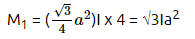(no. of turns = 4)
M2 = a2I × 3 = 3Ia2
(no. of turns = 3)

Q.7. In the product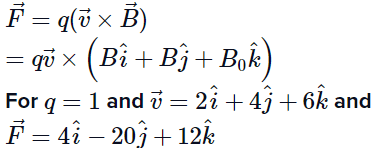What will be the complete expression for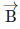?      (2021)
A: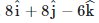B: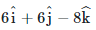C: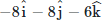D: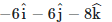Ans: D

Q.8. A spherical conductor of radius 10 cm has a charge of 3.2 × 10-7 C distributed uniformly. what is the magnitude of electric field at a point 15 cm from the centre of the sphere?      (2020)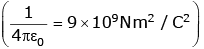Ans: D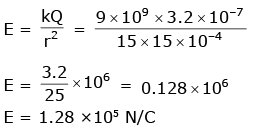Q.9. A long sol enoid of 50 cm length having 100 turns carries a current of 2.5 A. The magnetic field at the centre of the solenoid is : (μ0 = 4π × 10-7 Tm A-1)       (2020)
A: 6.28×10-5 T
B: 3.14×10-5 T
C: 6.28×10-4 T
D: 3.14×10-4 T
Ans:
C
Magnetic field for solenoid is given by
B = μ0 nI
n = N/ℓ
= 4π × 10-7 × 200 × 2.5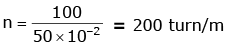= 4 × 3.14 × 10–7 × 500
= 2000 × 3.14 × 10–7
= 6.14 × 10–4 T

Q.10. A cylindrical conductor of radius R is carrying a constant current. The plot of the magnitude of the magnetic field. B with the distance d from the centre of the conductor, is correctly represented by the figure :      (2019)
A: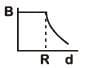B: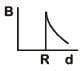C: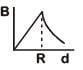D: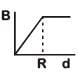Ans:
C
Solution: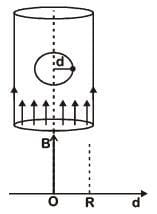Inside (d < R) Magnetic field inside conductor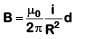or B = Kd   ...(i)
Straight line passing through origin
At surface (d = R)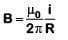...(ii)
Maximum at surface Outside (d > R)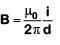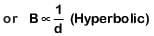Q.11. Ionized hydrogen atoms and α-particles with same momenta enters perpendicular to a constant magnetic field, B. The ratio of their radii of their paths rH : ra will be :     (2019)
A: 2 : 1
B: 1 : 2
C: 4 : 1
D: 1 : 4
Ans:
A
Solution: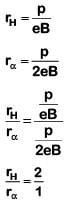Q.12. A 250-Turn rectangular coil of length 2.1 cm and width 1.25 cm carries a current of 85 µA and subjected to magnetic field of strength 0.85 T. Work done for rotating the coil by 180º against the torque is:-       (2017)
A: 4.55 µJ
B: 2.3 µJ
C: 1.15 µJ
D: 9.1 µJ
Ans:
D
Solution:

W = MB (cosθ1– cosθ2)
When it is rotated by angle 180º then
W = 2MB
W = 2 (NIA)B
= 2 x 250 x 85 x 10-6[1.25 x 2.1 x 10-4] x 85 x 10-2
= 9.1μJ

Q.13. An arrangement of three parallel straight wires placed perpendicular to plane of paper carrying same current 'I' along the same direction is shown in fig. Magnitude of force per unit length on the middle wire 'B' is given by :-      (2017)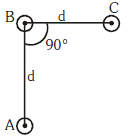A: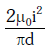B: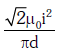C: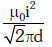D: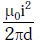Ans:
C
Solution: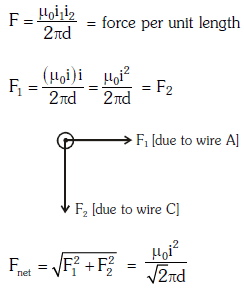Q.14. A long straight wire of radius a carries a steady current I. The current is uniformly distributed over its cross-section. The ratio of the magnetic fields B and B`, at a radial distances a/2 and 2a respectively, from the axis of the wire is:     (2016)
A: 4
B: 1/4
C: 1/2
D: 1
Ans:
D
Solution:

Consider two amperian loops of radius a/2 and 2a as shown in the diagram.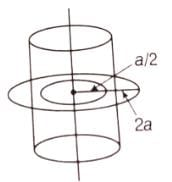Applying Ampere's circuital law for these loops, we get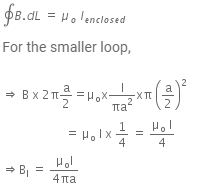B1 acts at a distance a/2 from the axis of the wire.
Similarly, for bigger amperian loop,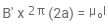Total current enclosed by amperian loop 2.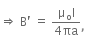at a distance 2a from the axis of the wire.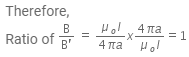Q.15. Two identical long conducting wires AOB and COD are placed at right angle to each other, with one above other such that ‘O’ is their common point for the two. The wires carry I1 and I2 currents, respectively. Point ‘P’ is lying at distance ‘d’ from ‘O’ along a direction perpendicular to the plane containing the wires. The magnetic field at the point ‘P’ will be :     (2014)
A: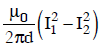B: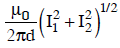C: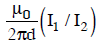D: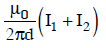Ans: B
Solution: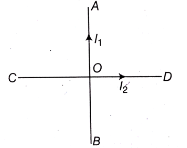The point P is lying at a distance d along the z-axis.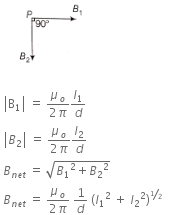The document NEET Previous Year Questions (2014-22): Moving Charges & Magnetism - Notes | Study Physics Class 12 - NEET is a part of the NEET Course Physics Class 12.
All you need of NEET at this link: NEET

## Physics Class 12

157 videos|452 docs|213 tests
 Use Code STAYHOME200 and get INR 200 additional OFF

## Physics Class 12

157 videos|452 docs|213 tests

### How to Prepare for NEET

Read our guide to prepare for NEET which is created by Toppers & the best Teachers

Track your progress, build streaks, highlight & save important lessons and more!

,

,

,

,

,

,

,

,

,

,

,

,

,

,

,

,

,

,

,

,

,

;